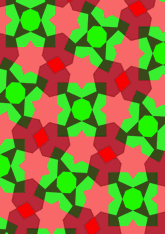# Bourgoin, Plate 170data12/P170

## Geometry

• The symmetry group of the tiling is 4*2 (p4g).
• All the internal angles of the constituent polygons are a multiple of 3.21 (π/56)°.
• Contains one regular two-pointed star polygon with vertex angle of 77.14 (3π/7)°.
• Contains one regular octagon.
• Contains one regular 7-pointed star polygon with vertex angle of 77.14 (3π/7)°.
• There are three non-regular reflective tiles.
• The tiling satisfies the interlace condition and has one finite interlace and one infinite interlace with straight cross-overs.
• The tiling is edge-to-edge.
• As drawn, contains about 193 polygons.

## References

Publications referenced: